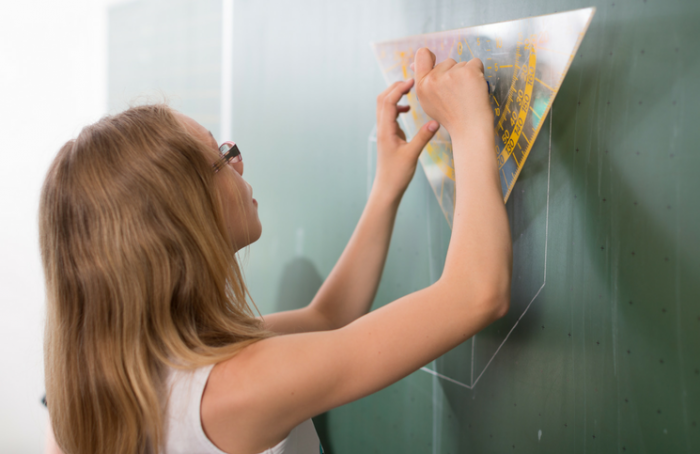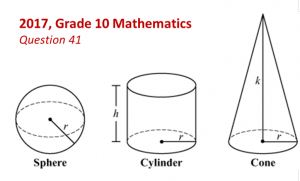The Formula Page is Your Friend – Improving Performance on MCAS Math

# The Formula Page is Your Friend – Improving Performance on MCAS Math## On MCAS, every point counts.

by Joan Reissman, Blended Learning Specialist

Although ELA has barely ended, MCAS math will be here before you know it on May 23 and 24 for high schools.

It seems obvious that the math formula page is there to help students, but few students really use it. Many questions, including open response items, are easy to solve if they just check their formulas. For example, the 2017 10th grade test had six questions that relied heavily on geometric formulas. There is almost always an open response question derived straight from the formula page. One of the best ways to show students the value of the formula page is to do one of these open response questions in class. And don’t forget the handy tool on the DESE website mentioned in my previous blog— the student work/scoring guides section. You’ll find it very useful for practicing open response.

Question 41 of the 2017 Grade 10 Mathematics test provides a good example of how the formula page can help students. The last thing you want them to do is not even attempt to answer the question. Always remind students that the first two parts of even the most difficult open response questions are relatively easy to answer. It’s also a good strategy to present your work neatly. While it’s true that readers are looking for the correct answer, a clear presentation with some mistakes will usually fare better than a sloppy one. Remember too that readers are looking at reasoning and a student will get partial credit for demonstrating conceptual understanding, even if there is a calculation error.

Take question 41 from the 2017 test, foundhere and project it. Make sure students have a copy of the formula page. Go over this question as a class. Students will see that parts (a) and (b) are easy to answer. If they plug in the values from the given formulas, they will get the correct answer. The third part takes some application of the geometric formulas and some algebraic manipulation. However, plugging in the values and isolating the variable are concepts that students have studied. The last part will be the most difficult. They will have to understand the relationship between the two formulas and do some algebraic manipulation to get the correct answer; but remind them that they still can get some points without completing the last part. And every point counts toward the final score.

Go over one example of each given response on the DESE website and have students decide what grade each deserves. You can find the student responses receiving 1 to 4 here. Show how making computation mistakes can still garner a score of 2. The student who received a 2 made math mistakes in all parts of the question, although she did use the correct formula in parts b and c and was close in part a. Then show your students the response that received a 3. Even the 3 response had math mistakes, but the student understood the most difficult part of the question. Finish with one of the responses that received a 4 so that students can see an answer that fulfills all parts of the question. You can repeat this exercise with similar questions from other years.

Analyzing varied responses and their scores will build students’ confidence in their ability to answer open response questions. On last year’s test, there were 6 questions that relied on the formulas page, including a short answer and an open response. Introduce your students to this page and help them get better scores. Don’t let them waste this opportunity to improve their performance. Remind them that on MCAS, every point counts.